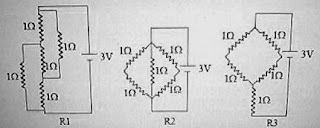## Wednesday, April 23, 2008

### IIT-JEE 2008 Questions on Direct Current Circuits

Two questions from direct current circuits appeared in the IIT-JEE 2008 question paper. Here are those questions:

Figure shows three resistor configurations R1, R2 and R3 connected to 3 V battery. If the power dissipated by the configurations R1, R2 and R3 are P1, P2 and P3 respectively, then(a) P1 > P2 > P3

(b) P1 > P3 > P2

(c) P2 > P1 > P3

(d) P3 > P2 > P1

For the configuration R1, the central 1 Ω resistor can be ignored since it is connected between equipotential points (similar to the galvanometer in a balanced Wheatstone bridge). The equivalent resistance connected across the battery is therefore 1 Ω. [2 Ω in parallel with 2 Ω].

The equivalent resistance in the configuration R2 is 0.5 Ω. [2 Ω, 2 Ω and 1 Ω in parallel]

The equivalent resistance in the configuration R3 is 2 Ω.

Since power P = V2/R we have P2 > P1 > P3 [Option (c)].

The second question which follows is Reasoning Type with 4 choices out of which only one is correct

STATEMENT-1

In a metre bridge experiment, null point null point for an unknown resistance is measured. Now, the unknown resistance is put inside an enclosure maintained at a higher temperature.. The null point can be obtained at the same point as before by decreasing the value of the standard resistanceand

STATEMENT-2

Resistance of a metal increases with increase in temperature.

(A) STATEMENT-1 is True, STATEMENT-2 is a correct explanation for STATEMENT-1

(B) STATEMENT-1 is True, STATEMENT-2 is True; STATEMENT-2 is NOT a correct explanation for STATEMENT-1

(C) STATEMENT-1 is True, STATEMENT-2 is False

(D) STATEMENT-1 is False, STATEMENT-2 is True

We have R/X = l1/l2

If the unknown resistance X is decreased on increasing its temperature, the null point can be obtained at the sme point as before by decreasing the value of the standard resistance. You should note that nothing is mentioned about the nature of the unknown resistance. It can be a negative temperature coefficient (of resistance) device such as a thermistor. So, statement 1 is true.

Metals have positive temperature coefficient of resistance. So, statement 2 is true. But it is not a correct explanation for statement-1.

The correct option is (B).

[If the unknown resistance is a metallic wire such as copper wire or nichrome wire, the resistance will increase on heating and the correct option will then be (D).